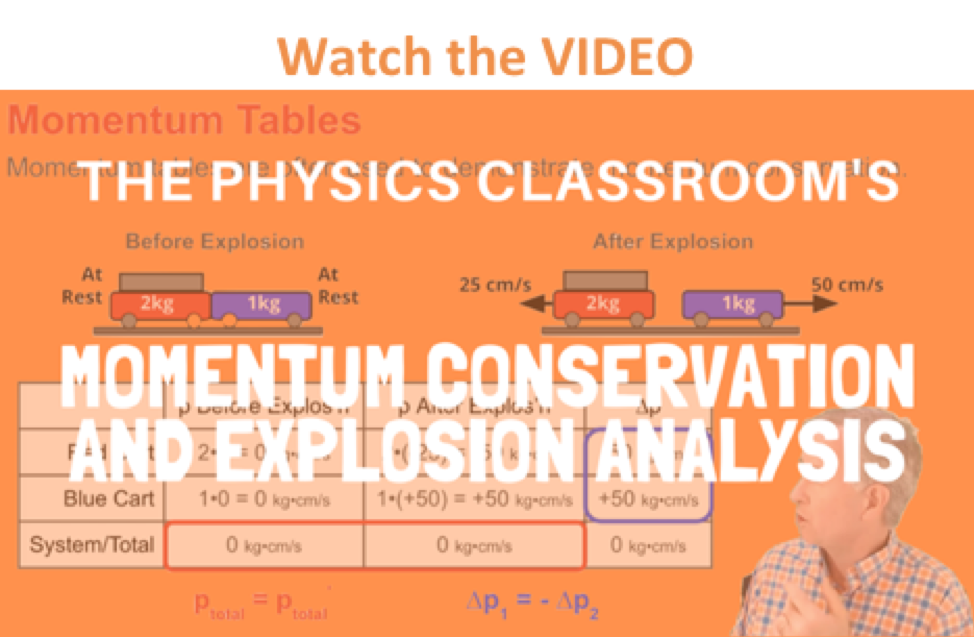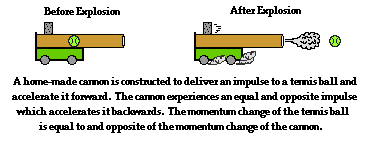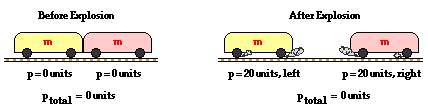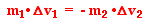Momentum and Its Conservation - Lesson 2 - The Law of Momentum Conservation

# Momentum Conservation in ExplosionsAs discussed in a previous part of Lesson 2, total system momentum is conserved for collisions between objects in an isolated system. For collisions occurring in isolated systems, there are no exceptions to this law. This same principle of momentum conservation can be applied to explosions. In an explosion, an internal impulse acts in order to propel the parts of a system (often a single object) into a variety of directions. After the explosion, the individual parts of the system (that is often a collection of fragments from the original object) have momentum. If the vector sum of all individual parts of the system could be added together to determine the total momentum after the explosion, then it should be the same as the total momentum before the explosion. Just like in collisions, total system momentum is conserved.Momentum conservation is often demonstrated in a Physics class with a homemade cannon demonstration. A homemade cannon is placed upon a cart and loaded with a tennis ball. The cannon is equipped with a reaction chamber into which a small amount of fuel is inserted. The fuel is ignited, setting off an explosion that propels the tennis ball through the muzzle of the cannon. The impulse of the explosion changes the momentum of the tennis ball as it exits the muzzle at high speed. The cannon experienced the same impulse, changing its momentum from zero to a final value as it recoils backwards. Due to the relatively larger mass of the cannon, its backwards recoil speed is considerably less than the forward speed of the tennis ball.In the exploding cannon demonstration, total system momentum is conserved. The system consists of two objects - a cannon and a tennis ball. Before the explosion, the total momentum of the system is zero since the cannon and the tennis ball located inside of it are both at rest. After the explosion, the total momentum of the system must still be zero. If the ball acquires 50 units of forward momentum, then the cannon acquires 50 units of backwards momentum. The vector sum of the individual momenta of the two objects is 0. Total system momentum is conserved.

As another demonstration of momentum conservation, consider two low-friction carts at rest on a track. The system consists of the two individual carts initially at rest. The total momentum of the system is zero before the explosion. One of the carts is equipped with a spring-loaded plunger that can be released by tapping on a small pin. The spring is compressed and the carts are placed next to each other. The pin is tapped, the plunger is released, and an explosion-like impulse sets both carts in motion along the track in opposite directions. One cart acquires a rightward momentum while the other cart acquires a leftward momentum. If 20 units of forward momentum are acquired by the rightward-moving cart, then 20 units of backwards momentum is acquired by the leftward-moving cart. The vector sum of the momentum of the individual carts is 0 units. Total system momentum is conserved.### Equal and Opposite Momentum Changes

Just like in collisions, the two objects involved encounter the same force for the same amount of time directed in opposite directions. This results in impulses that are equal in magnitude and opposite in direction. And since an impulse causes and is equal to a change in momentum, both carts encounter momentum changes that are equal in magnitude and opposite in direction. If the exploding system includes two objects or two parts, this principle can be stated in the form of an equation as:If the masses of the two objects are equal, then their post-explosion velocity will be equal in magnitude (assuming the system is initially at rest). If the masses of the two objects are unequal, then they will be set in motion by the explosion with different speeds. Yet even if the masses of the two objects are different, the momentum change of the two objects (mass • velocity change) will be equal in magnitude.

The diagram below depicts a variety of situations involving explosion-like impulses acting between two carts on a low-friction track. The mass of the carts is different in each situation. In each situation, total system momentum is conserved as the momentum change of one cart is equal and opposite the momentum change of the other cart.In each of the above situations, the impulse on the carts is the same - a value of 20 kg•cm/s (or cN•s). Since the same spring is used, the same impulse is delivered. Thus, each cart encounters the same momentum change in every situation - a value of 20 kg•cm/s. For the same momentum change, an object with twice the mass will encounter one-half the velocity change. And an object with four times the mass will encounter one-fourth the velocity change.

### Solving Explosion Momentum Problems

Since total system momentum is conserved in an explosion occurring in an isolated system, momentum principles can be used to make predictions about the resulting velocity of an object. Problem solving for explosion situations is a common part of most high school physics experiences. Consider for instance the following problem:

A 56.2-gram tennis ball is loaded into a 1.27-kg homemade cannon. The cannon is at rest when it is ignited. Immediately after the impulse of the explosion, a photogate timer measures the cannon to recoil backwards a distance of 6.1 cm in 0.0218 seconds. Determine the post-explosion speed of the cannon and of the tennis ball.

Like any problem in physics, this one is best approached by listing the known information.

Given:

 Cannon: m = 1.27 kg d = 6.1 cm t = 0.0218 s
 Ball: m = 56.2 g = 0.0562 kg

The strategy for solving for the speed of the cannon is to recognize that the cannon travels 6.1 cm at a constant speed in the 0.0218 seconds. The speed can be assumed constant since the problem states that it was measured after the impulse of the explosion when the acceleration had ceased. Since the cannon was moving at constant speed during this time, the distance/time ratio will provide a post-explosion speed value.

vcannon = d / t = (6.1 cm) / (0.0218 s) = 280 cm/s (rounded)

The strategy for solving for the post-explosion speed of the tennis ball involves using momentum conservation principles. If momentum is to be conserved, then the after-explosion momentum of the system must be zero (since the pre-explosion momentum was zero). For this to be true, then the post-explosion momentum of the tennis ball must be equal in magnitude (and opposite in direction) of that of the cannon. That is,

mball • vball = - mcannon • vcannon

The negative sign in the above equation serves the purpose of making the momenta of the two objects opposite in direction. Now values of mass and velocity can be substituted into the above equation to determine the post-explosion velocity of the tennis ball. (Note that a negative velocity has been inserted for the cannon's velocity.)

(0.0562 kg) • vball = - (1.27 kg) • (-280 cm/s)

vball = - (1.27 kg) • (-280 cm/s) /  (0.0562 kg)

vball = 6323.26 cm/s

vball = 63.2 m/s

Using momentum explosion, the ball is propelled forward with a speed of 63.2 m/s - that's 141 miles/hour!

It's worth noting that another method of solving for the ball's velocity would be to use a momentum table similar to the one used previously in Lesson 2 for collision problems. In the table, the pre- and post-explosion momentum of the cannon and the tennis ball. This is illustrated below.

 Momentum Before Explosion Momentum After Explosion Cannon 0 (1.27 kg) • (-280 cm/s) = -355 kg•cm/s Tennis Ball 0 (0.0562 kg) • v Total 0 0

The variable v is used for the post-explosion velocity of the tennis ball. Using the table, one would state that the sum of the cannon and the tennis ball's momentum after the explosion must sum to the total system momentum of 0 as listed in the last row of the table. Thus,

-355 kg•cm/s + (0.0562 kg) • v = 0

Solving for v yields 6323 cm/s or 63.2 m/s - consistent with the previous solution method.

Using the table means that you can use the same problem solving strategy for both collisions and explosions. After all, it is the same momentum conservation principle that governs both situations. Whether it is a collision or an explosion, if it occurs in an isolated system, then each object involved encounters the same impulse to cause the same momentum change. The impulse and momentum change on each object are equal in magnitude and opposite in direction. Thus, the total system momentum is conserved.

### We Would Like to Suggest ...Sometimes it isn't enough to just read about it. You have to interact with it! And that's exactly what you do when you use one of The Physics Classroom's Interactives. We would like to suggest that you combine the reading of this page with the use of our Exploding Carts Interactive and/or our Collision Carts Ineractive. Both Interactives can be found in the Physics Interactive section of our website and provide an interactive experience in analyzing the momentum of individual objects and systems of objects in collisions.

1. Two pop cans are at rest on a stand. A firecracker is placed between the cans and lit. The firecracker explodes and exerts equal and opposite forces on the two cans. Assuming the system of two cans to be isolated, the post-explosion momentum of the system ____.

a. is dependent upon the mass and velocities of the two cans

b. is dependent upon the velocities of the two cans (but not their mass)

c. is typically a very large value

d. can be a positive, negative or zero value

e. is definitely zero

2. Students of varying mass are placed on large carts and deliver impulses to each other's carts, thus changing their momenta. In some cases, the carts are loaded with equal mass; in other cases they are unequal. In some cases, the students push off each other; in other cases, only one team does the pushing. For each situation, list the letter of the team that ends up with the greatest momentum. If they have the same momentum, then do not list a letter for that situation. Enter the four letters (or three or two or ...) in alphabetical order.3. Two ice dancers are at rest on the ice, facing each other with their hands together. They push off on each other in order to set each other in motion. The subsequent momentum change (magnitude only) of the two skaters will be ____.

a. greatest for the skater who is pushed upon with the greatest force

b. greatest for the skater who pushes with the greatest force

c. the same for each skater

d. greatest for the skater with the most mass

e. greatest for the skater with the least mass

4. A 62.1-kg male ice skater is facing a 42.8-kg female ice skater. They are at rest on the ice. They push off each other and move in opposite directions. The female skater moves backwards with a speed of 3.11 m/s. Determine the post-impulse speed of the male skater.

5. A 1.5-kg cannon is mounted on top of a 2.0-kg cart and loaded with a 52.7-gram ball. The cannon, cart, and ball are moving forward with a speed of 1.27 m/s. The cannon is ignited and launches a 52.7-gram ball forward with a speed of 75 m/s. Determine the post-explosion velocity of the cannon and cart.